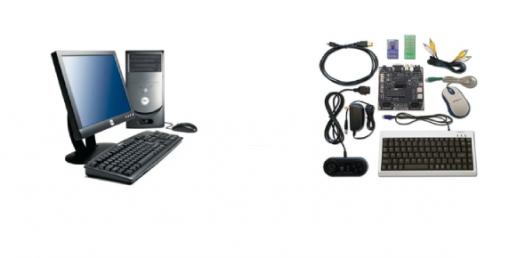# Computer Quiz: Hardware Parts! Trivia Questions

13 Questions | Attempts: 941SettingsParts of a computer quiz

• 1.
1,000 characters are equal to what? (2 correct answers)
• A.

1,000 bytes

• B.

1 kilobyte

• C.

1 gigabyte

• D.

1 megabyte

• 2.
What memory is Non-Volatile.(Hint: Programs related to operating system are stored here.)
• 3.
What is used for encoding alphabet?
• A.

Bits

• B.

Barts

• C.

Bytes

• D.

Bakes

• 4.
What type is this included in?-Wordprocessing-Spreadsheets-Games-DPT
• A.

Hardware

• B.

Software

• 5.
1,000 megabytes =
• A.

1,000 gigibytes

• B.

1 gigibyte

• C.

1,000 kilobytes

• D.

1 gigabyte

• 6.
1,000 kilobytes =
• A.

1 byte

• B.

1,000 gigabytes

• C.

1 megabytes

• D.

1,000 megabytes

• 7.
What does RAM stand for?
• 8.
How many bits to make a byte?
• A.

8 bits

• B.

14 bits

• C.

5 bits

• D.

7 bits

• E.

10 bits

• 9.
What are these examples of?-Printer-Mouse-Moniter-Keyboard-Scanner
• 10.
Give me a characteristic of Hardware.
• 11.
What is the Binary Code?
• A.

2 and 3

• B.

4 and 5

• C.

77 and 99

• D.

6 and 7

• E.

0 and 1

• 12.
The CPU is also known as the what of the computer.
• 13.
What is the speed of the CPU?
• A.

Gerghertz

• B.

Megahertz

• C.

Bibahertz

• D.

Kilohertz

## Related TopicsBack to top
×

Wait!
Here's an interesting quiz for you.